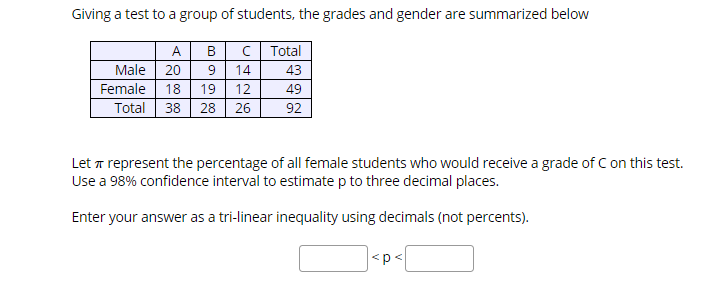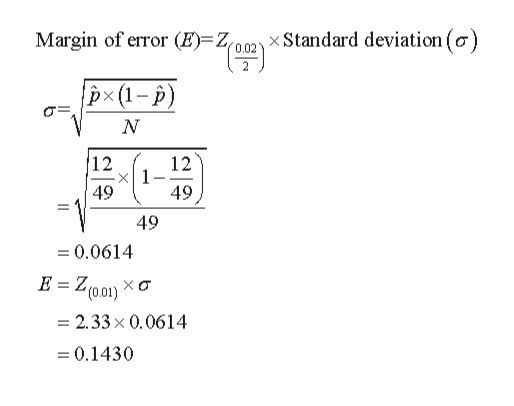# Giving a test to a group of students, the grades and gender are summarized belowTotalA.ВCMale201443Female191249Total 38282692Let T represent the percentage of all female students who would receive a grade of C on this test.Use a 98% confidence interval to estimate p to three decimal places.Enter your answer as a tri-linear inequality using decimals (not percents).

Question
165 viewshelp_outlineImage TranscriptioncloseGiving a test to a group of students, the grades and gender are summarized below Total A. В C Male 20 14 43 Female 19 12 49 Total 38 28 26 92 Let T represent the percentage of all female students who would receive a grade of C on this test. Use a 98% confidence interval to estimate p to three decimal places. Enter your answer as a tri-linear inequality using decimals (not percents).
check_circle

Step 1

It is given that total number of females (N) is 49. Total number of females who get C grade (n) is 19.

Thus,

The probability that a female gets C grade, can be calculated as:

Step 2

Check the condition of normality,

if Np and Np(1-p) both are greater than 5, then it will follow normality.

Step 3

Further,...help_outlineImage TranscriptioncloseMargin of eror (E)=2o02) x Standard deviation (a) px(1-p) 12 12 -X 1 49 49 49 =0.0614 (0.01) 2.33x 0.0614 =0.1430 fullscreen

### Want to see the full answer?

See Solution

#### Want to see this answer and more?

Solutions are written by subject experts who are available 24/7. Questions are typically answered within 1 hour.*

See Solution
*Response times may vary by subject and question.
Tagged in

### Other